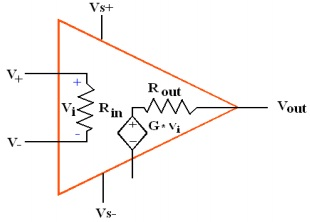Op-amp Integrator

Objective

To design and study an Integrator using Op-amp 741.

Introduction

The Integrator is a circuit using Op-amp that performs the mathematical operation of Integration. The integrator acts like a storage element that "produces a voltage output which is proportional to the integral of its input voltage with respect to time". In other words the magnitude of the output signal is determined by the length of time a voltage is present at its input as the current through the feedback loop charges or discharges the capacitor as the required negative feedback occurs through the capacitor.

An operational Amplifier, often called an Op-amp, is a DC-coupled high-gain electronic voltage amplifier with differential inputs ad usually a single output. Typically the output of the Op-amp is controlled either by negative feedback, which largely determines the magnitude of its output voltage gain, or by positive feedback, which facilitates regenerative gain and oscillation. High input impedance at the input terminals and low output impedance are important typical characteristics.

Ideal Op-amp: The figure below shows an example of an ideal operational amplifier. The main part in an amplifier is the dependent voltage source that increases in relation to the voltage drop across Rin, thus amplifying the voltage difference between V + and V -. Many uses have been found for Op-amp and an ideal Op-amp seeks to characterize the physical phenomena that make Op-amps useful.Fig.1 Ideal Op-amp

Vs+ and Vs- are not connected to the circuit within the Op-amp because they power the dependent voltage source’s circuit. These are notable, however, because they determine the maximum voltage the dependent voltage source can output. For any input voltage the ideal Op-amp has,
a. Infinite open-loop gain
b. Infinite bandwidth
c. Infinite input impedance
d. Zero offset voltage
e. Infinite slew rate
f. Zero output impedance and
g. Zero noise